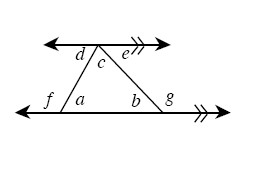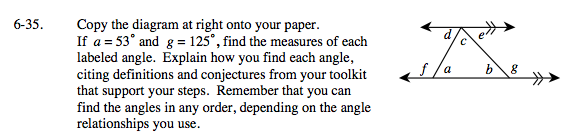Home > GC > Chapter 6 > Lesson 6.1.4 > Problem6-35

6-35.Copy the diagram at right onto your paper. If a = 53° and g = 125°, find the measures of each labeled angle. Explain how you find each angle, citing definitions and conjectures from your toolkit that support your steps. Remember that you can find the angles in any order, depending on the angle relationships you use. Homework Help ✎You can find the angles in any order.

Since ∠a and ∠f are adjacent supplementary angles (linear pair), then we can solve this algebraically.
ma + mf = 180°
53° + mf = 180°
mf = 27°

b and ∠g are another linear pair.
They also are supplementary.
mb + mg = 180°
mb + 125° = 180 °
mb = 55°

If parallel lines are cut by a transversal, then alternate interior angles are congruent.

ma = md = 53°
mb = me = 55°

Using the Triangle Angle Sum theorem:
ma + mb + mc = 180°
53° + 55° + mc = 180°
mc = 72°

a = 53°, b = 55°, c = 72°, d = 53°, e = 55°, f = 127°, g = 125°